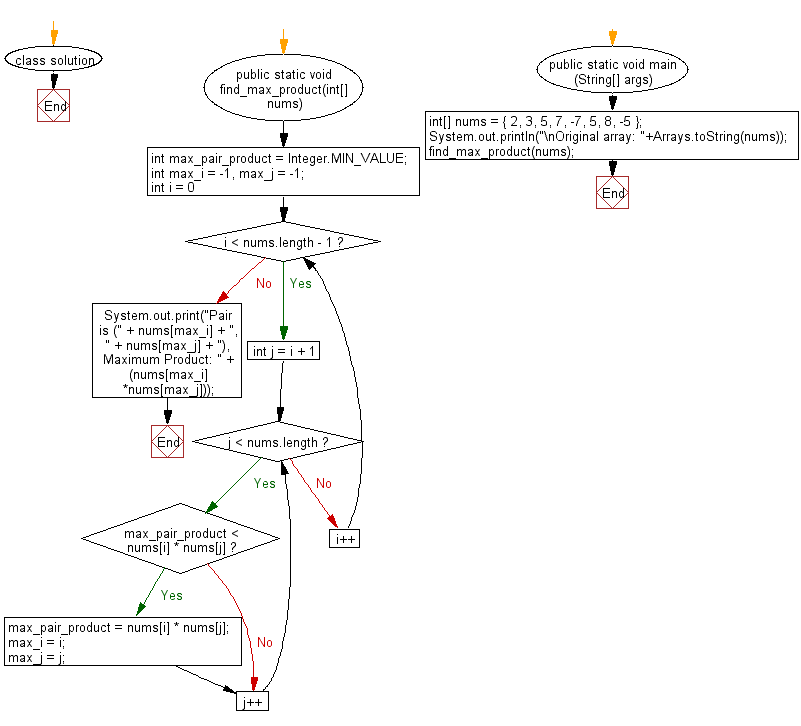﻿ Java: Find maximum product of two integers in an array# Java Array Exercises: Find maximum product of two integers in a given array of integers

## Java Array: Exercise-59 with Solution

Write a Java program to find maximum product of two integers in a given array of integers.

Example:
Input :
nums = { 2, 3, 5, 7, -7, 5, 8, -5 }
Output:
Pair is (7, 8), Maximum Product: 56

Sample Solution:

Java Code:

``````import java.util.*;
class solution
{
public static void find_max_product(int[] nums)
{
int max_pair_product = Integer.MIN_VALUE;
int max_i = -1, max_j = -1;

for (int i = 0; i < nums.length - 1; i++)
{
for (int j = i + 1; j < nums.length; j++)
{
if (max_pair_product < nums[i] * nums[j])
{
max_pair_product = nums[i] * nums[j];
max_i = i;
max_j = j;
}
}
}

System.out.print("Pair is (" + nums[max_i] + ", " + nums[max_j] + "), Maximum Product: " + (nums[max_i]*nums[max_j]));
}

public static void main (String[] args)
{
int[] nums = { 2, 3, 5, 7, -7, 5, 8, -5 };
System.out.println("\nOriginal array: "+Arrays.toString(nums));
find_max_product(nums);
}
}
```
```

Sample Output:

```Original array: [2, 3, 5, 7, -7, 5, 8, -5]
Pair is (7, 8), Maximum Product: 56
```

Flowchart:Java Code Editor:

Improve this sample solution and post your code through Disqus

What is the difficulty level of this exercise?

Test your Programming skills with w3resource's quiz.

﻿

## Java: Tips of the Day

countOccurrences

Counts the occurrences of a value in an array.

Use Arrays.stream().filter().count() to count total number of values that equals the specified value.

```public static long countOccurrences(int[] numbers, int value) {
return Arrays.stream(numbers)
.filter(number -> number == value)
.count();
}
```

Ref: https://bit.ly/3kCAgLb